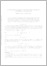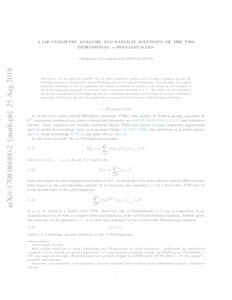# A Lie symmetry analysis and explicit solutions of the two-dimensional ∞-Polylaplacian

Papamikos, Georgios and Pryer, Tristan (2019) 'A Lie symmetry analysis and explicit solutions of the two-dimensional ∞-Polylaplacian.' Studies in Applied Mathematics, 142 (1). 48 - 64. ISSN 0022-2526Preview
Text
A Lie symmetry analysis and explicit solutions of the two dimensional inf-polylaplacian.pdf - Accepted Version

Download (243kB) | Preview

## Abstract

In this work, we consider the Lie point symmetry analysis of a strongly nonlinear partial differential equation of third order, the ∞‐Polylaplacian, in two spatial dimensions. This equation is a higher order generalization of the ∞‐Laplacian, also known as Aronsson's equation, and arises as the analog of the Euler–Lagrange equations of a second‐order variational principle in L∞. We obtain its full symmetry group, one‐dimensional Lie subalgebras and the corresponding symmetry reductions to ordinary differential equations. Finally, we use the Lie symmetries to construct new invariant ∞‐Polyharmonic functions.

Item Type: Article Faculty of Science and Health > Mathematical Sciences, Department of Elements 05 Nov 2020 13:30 05 Nov 2020 13:30 http://repository.essex.ac.uk/id/eprint/26459

### Actions (login required)View Item